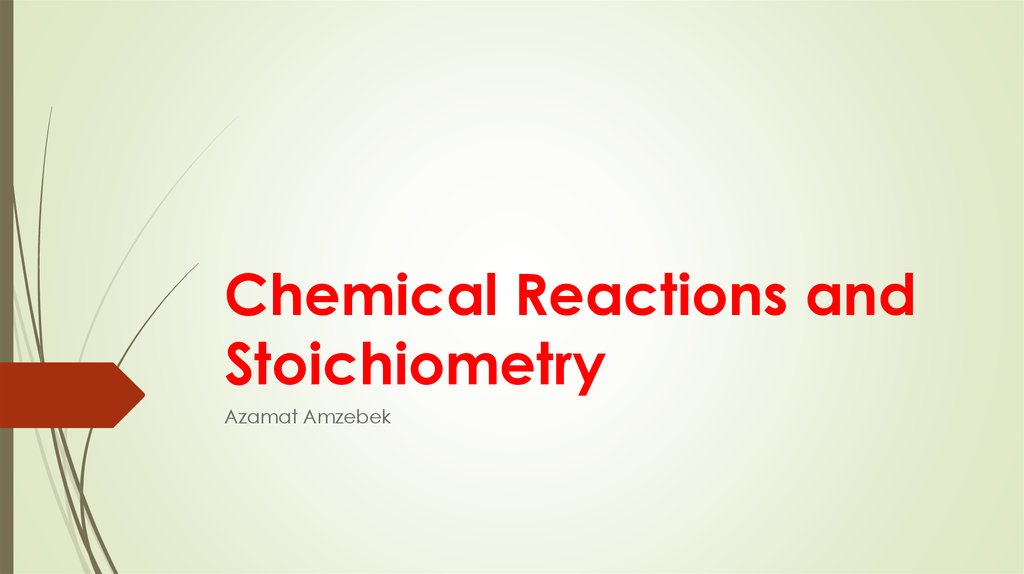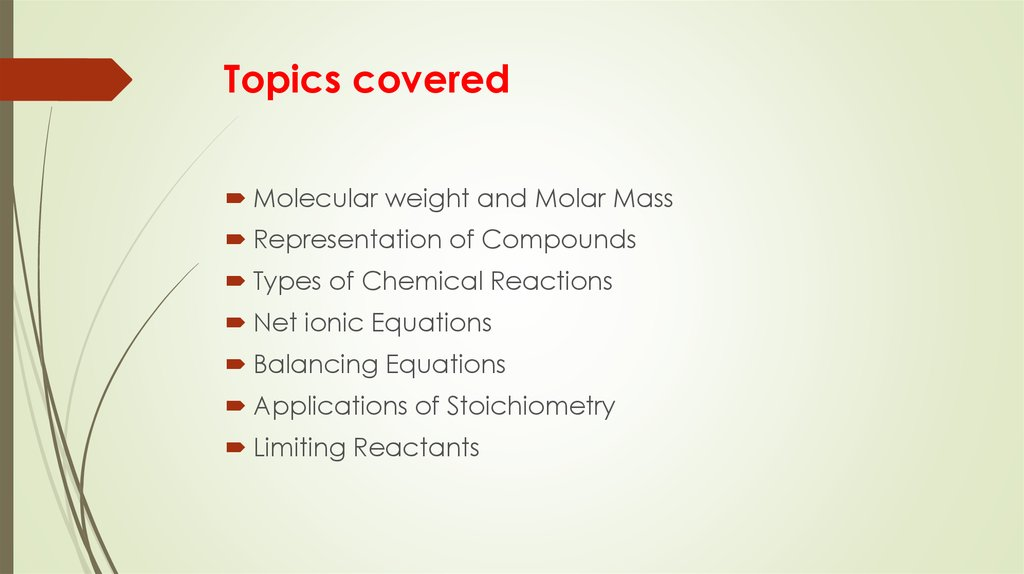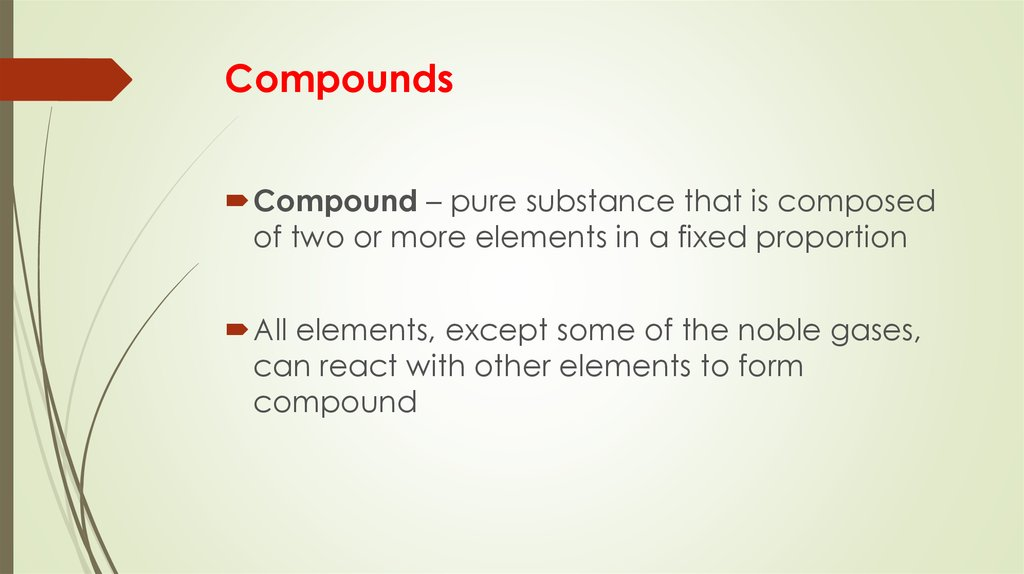# Chemical Reactions and Stoichiometry

Azamat Amzebek

## 2. Topics covered

Molecular weight and Molar Mass
Representation of Compounds
Types of Chemical Reactions
Net ionic Equations
Balancing Equations
Applications of Stoichiometry
Limiting Reactants

## 3. Compounds

Compound – pure substance that is composed
of two or more elements in a fixed proportion
All elements, except some of the noble gases,
can react with other elements to form
compound

## 4. Molecular weight and Molar mass

A molecule is a combination of two or more
atoms held together by covalent bonds.
The molecular weight is simply the sum of the
weights of the atoms that make up the
molecule
MOLAR MASS = MOLECULAR WEIGHT
Number of moles = weight of sample (g) / molar mass(g/mol)

## 5.

Representation of compounds
Law of Constant Composition – any sample of a
given compound will contain the same
elements in the identical mass ratio
Empirical formula gives the simplest whole
number ratio of the elements in the compound.
The molecular formula gives the exact number
of atoms of each element in a molecule of the
compound

## 6. Percent composition

It is the weight percent of the element in a
specific compound.

## 7. Types of chemical reactions

1. Synthesis Reactions
Two or more reactants form one product

## 8. Types of chemical reactions

2. Decomposition Reactions
One in which a compound breaks down into
two or more substances, usually as a result of
heating

## 9. Types of chemical reactions

3. Single Displacement Reactions
An atom of one compound is replaced by an
atom of another element

## 10. Types of Chemical Reactions

4. Double Displacement Reactions
Elements from two different compounds
displace each other to form two new
compounds

## 11. Net Ionic Equations

Because reactions such as displacements often
involve ions in a solution, they can be written in
ionic form
Very important to demonstrate the actual
reaction occurring

## 12. Balancing Equations

From the law of conservation of mass, the mass
of the reactants in a reaction must be equal to
the mass of the products
Stoichiometry is essentially the study of how the
quantities of reactants and products are related
in a chemical reaction.

## 15. Limiting Reactants

Limiting reactant limits the amounts of product
that can be formed in the reaction
The reactant that remains after all of the limiting
reactant is used up is called the excess reactant

## 16. Yields

The yield of a reaction is the amount of product
predicted or obtained when the reaction is
carried out.